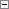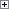Copies one or more elements in an array to a new array.

COPYARRAY(NewArray, Array, Position[, Length])

Parameters

NewArray

Type: Array

The array to copy to; the destination array.

Array

Type: Array

The array to copy from; the source array.

Position

Type: Integer

The position of the first array element to copy.

Length

Type: Integer

The number of array elements to copy. If you do not specify Length, all array elements are copied from Position to the last element. Use the following equation to determine valid values.

1 =< LENGTH =< MAXLEN(ARRAY) - POSITION + 1Remarks

You can only copy from one-dimensional arrays. Repeat the COPYARRAY function to copy two-dimensional and three-dimensional arrays.

You cannot copy an array if the data type of the array is a complex data type. For more information about complex data types, see C/AL Data Types.Example

The following example assigns values to Array1 and copies values from Array1 to Array2. Array1 is an integer array with the Dimensions Property set to 10. It contains integers from 1 to 10. The example code copies the numbers 6, 7, 8, 9, and 10 to Array2, an integer array with the Dimensions property set to 5. This example requires that you create the following variables in the C/AL Globals window.

Variable name DataType Dimension

Array1

Integer

10

Array2

Integer

5Copy Code
Array1 := 1;
Array1 := 2;
Array1 := 3;
Array1 := 4;
Array1 := 5;
Array1 := 6;
Array1 := 7;
Array1 := 8;
Array1 := 9;
Array1 := 10;
COPYARRAY(Array2,Array1,6,5);

If Array1 is an integer array with dimension 10, and it contains the numbers from 1 to 10, and Array2 is an integer array with dimension 5, then the following command causes a run-time error.Copy Code
COPYARRAY(Array2,Array1,3);

The error occurs because the code attempts to copy eight elements from Array1 to Array2, and Array2 has room for only five elements.